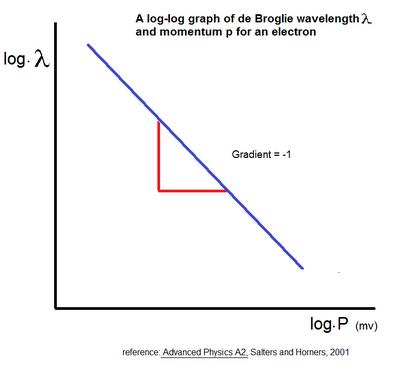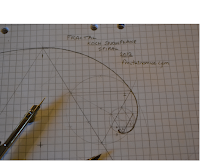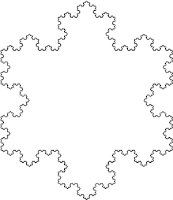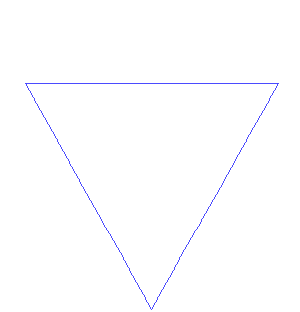## Posts

Showing posts from 2012

### Koch Snowflake and Fibonacci

This entry is to state that I looked at whether the Koch Snowflake has a Fibonacci number in it: for some reason I did not publish it. In the earlier entry 'the  Fractal Multiplier ' I noticed that the total area is 1.6 times the area of the first triangle. It is of interest to me (the author), and of my mathematician colleague’s, that the Koch snowflake fractal multiplier is 1.6. This 1.6 is very close to (but not the same as!) the  Fibonacci or Golden ratio of 1.618 .

### Fractal Log Analysis Linear functionsKoch Snowflake Fractal Log Analysis These are diagrams that I created during the last summer, I hoped that they would shed some light on fractal elasticity: they didn't. But, in saying that, I am not finished yet with them, I don't have the time, or the deeper knowledge to do a full analysis with them.  I am publishing them to show they exist and to show that this is what I have been doing, and because better to have them here, than still on my computer. Maybe someone else can look at them, and make something of them.  I am sure -   and can therefore  infer -  from the shape, and characteristics of them in  this analysis , t hat this  is the origin of  the classical  linear demand functions,  and linear supply functions - and all this from an understanding of the fractal.  I can only think of the: 'walk like a duck, quacks like a duck'. Linear Area Function, derived from the Koch Snowflake fractal.

### Measuring knowledge elasticity with Youtube

Measuring knowledge elasticity with Youtube  A quick note on something I thought of some time ago. Many Youtube clips are not shown in their entirety,  as one clip, but as series of  clips of (around) 10 minute. It has been interesting to me to note the number of  'views' for each of these 10 minute clips. One might think that the counts should be the same for each, but they are not. Are these counts a measure of the value of the knowledge in the clip. If the numbers remain near constant, one might say the knowledge is elastic - more knowledge is to be gained by watching the next. If the numbers diminish, one might say the knowledge is inelastic - more knowledge is not gained by an watching another.

### The Fractal Cat

The fractal cat: as opposed to the quantum cat A discussion entry. At what size (or scale) would I have to shrink to before my cat would eat me? Venus is my cat, and is nicest- calmest cat you can think of, but I have seen her eat mice - not pretty. Is our relationship all about scale? And is this scale a measure, or determinant of power.

### Fractal Time: Absolute or Relative?

This is a discussion entry: based on my fractal discoveries.   I have since published in 2023:  The fractal with no observation demonstrates no time.  T he fractal  with observation   demonstrates the passing of time, but not absolute time, but relative time. 1. No time: -  A fractal in an isolated superposition demonstrates no time. It is not until a reference point is provided, an observation is made - that time is time. When we have a reference point on the fractal, we 'know' position.  The modern clock itself may be a reference point - without it, we could be anywhere, or at any time. Without it, we are lost, we are in the chaos. The importance of a reference in time is just as important as any other reference  –  it is to 'know'. Absolute time: Recently I in this blog I have been exploring two key areas of science in terms of the fractal: the expanding fractal (universe), and the de Broglie wave function. In both of these entries I have had to action so

### Demand curve and de Broglie wavefunctionDemand curve and the de Broglie wave function    I have since published in 2023:  This entry has been hanging over me for some time, and I never published it earlier because I never thought I had it quite right, or it never felt complete. Though those feelings have not changed, I have (now) decided to publish what I have, intending that my theory will kindle interest and discussion to further develop it. What really is a demand curve anyway? Do they really exist? The reality may be that they are not a real physical, tangible object, but instead, show the possibilities of goods and services in terms of price and quantity; as with quantum theory, to produce such a curve invokes the ‘measurement’ problem . It is as if the demand curve is a superposition of all the possible outcomes, just as in quantum mechanics. My hypothesis: The de Broglie wave function and the (consumer) demand function and corresponding curve, are both different manifestations of the same thing. This theo

### The expanding fractalThe expanding fractal Update 2014 09 22 Yesterday I published/posted at  academia.edu  : Fractal Geometry a Possible Explanation to the Accelerating Expansion of the Universe and Other Standard ΛCMB Model Anomalies also at:   http://vixra.org/abs/1503.0232 Abstract One of the great questions in modern cosmology today is what is causing the accelerating expansion of the universe – the so called dark energy. It has been recently discovered this property is not unique to the universe; trees also do it and trees are fractals. Do fractals offer insight to the accelerating expansion a property of the universe and more? In this investigation a simple experiment was undertaken on the classical (Koch snowflake) fractal. It was inverted to model and record observations from within an iterating fractal set as if at a static (measured) position. New triangles sizes were held constant allowing earlier triangles in the set to expand as the set iterated. Velo

### Fractal Koch Snowflake SpiralThe Koch Snowflake Spiral On route to understanding, if  fractals have a 'wavy' like nature , I finally put pencil to paper and drew what I'd been thinking. I have been pondering on what effect a 'mutation' or change to one triangle - a dot at the apex of the triangle as shown below - would have or show on the  iterating  Koch Snowflake. I envisaged that it would spiral to infinity as shown below. By tracing a smooth curve through the (red) 'dots' series of iterated mutant triangles, I would develop - what looks like - a kind of logarithmic spiral , or a wave or pulse if this spiral curve was viewed in the front elevation view as opposed to the plan elevation (above). Method As a circle's radius (what the compass is set to) scribes  6 times around the circles circumference , the three corners of the 1st equilateral triangle (iteration 1) can be produced, and the apex of the 2nd triangle (iteration 2) can also be marked. With the compass sti

### Fractal: MultiplierDevelopment and growth of the fractal demonstrate the ( money and Keynesian) Multiplier. The (Keynesian) Multiplier shows how an initial injection of expenditure into an economic system goes on to create more income. This is because added expenditure – in itself – sets off additional rounds of expenditure with each and every hand or round this income passes. This principle of 'multiplying' the initial injection can be demonstrated by the use of the fractal. In the diagram below: income is represented by the area of each and every triangle, and the rounds of spending by every iteration of the rule.     The initial injection (iteration 1) is represented by the first triangle – which has an arbitrary area of 1. The next round adds 3 extra triangles, increasing the total area of the snowflake. This (principle) process continues until the changes in the area of the triangle  – after  each iteration  –  change the total area of the snowflake no more. In the diagram ab

### Fractal Laws of information

I have published - as a page -  the Laws of Information ; all of which  I have collected, and derived from the fractal. Like a fractal itself, these laws will/should - over time - grow, develop and form shape .

### Fractal SpeedI have since published in 2023:  What is the maximum speed a fractal can be produced?   The fractal is produced at the fractal processing speed (fractal speed), this is the speed at which a  discernible  fractal  shape can be created. This speed also determines the speed of zoom – or magnification into the fractal. It is also the speed of the fractal – wave. Fractal zoom Fractal speed can be demonstrated by drawing the Koch snowflake freehand. This is rather slow and timely ; a much faster method is with modern computers as shown below. The speed is thus limited by the processing power of production. I have published early entries on the production of the fractal. The average modern computer (in 2011) cannot produce many more than 7 iterations  –  in one view or 'fractal paradigm'  – before the computer crashes. To produce more, or see more, we must zoom – forward and into and fractal. The maximum zoom fractal speed must be 'Maxwell’s'  – speed of light

### Fractal UncertaintyI have since published in 2023:  (Fractal)  Quantum Uncertainty Observing a (Koch Snowflake) fractal (Fig. 1 below) in superposition : p osition, scale and direction (of growth) of any one triangle, is only ever known with reference to another reference point – another measurement or observation – and since there is no reference to be found in this state of ceteris paribus or isolation, there is only absolute uncertainly – of the above. In a previous entry – fractal ceteris paribus – I explained this fractal feature – independent of any knowledge of quantum theory. Can the position be determined - in the above fractal animations?

### Fractal: Wave Particle DualityI have since published in 2023:  This entry is one of a set of entries on the fractal and the strange (quantum-like) nature of them. I use the word quantum because there is no other area of knowledge that comes close to explaining or relating to the discoveries I am making with fractal geometry. Blair 11,03,2013 Wave and particle D uality - and the fractal The below entry is a discovery, not an explanation.  I (intend to) write what I see, and what I expect I have found - I do not pretend to fully understand. Just as the atom can ' weirdly' be described as being both a particle and as a ‘smeared out’ wave at the same time, so too, as I shall demonstrate, can the fractal be described in such a way  - only for the fractal it is not so weird. The fractal demonstrating a (discrete) particle: The fractal is defined by  a pattern, object or shape  repeating or iterating. The Koch Snowflake (below) demonstrates this iterating - the triangle represents the particle.

### Fractal EntanglementThis entry I hope adds to the discussion on quantum entanglement. Fractal Entanglement The fractal at a state of fractal superposition, and in perfect isolation – with no interference from other fractals – may demonstrate the principle of (quantum) entanglement.   The 'general' fractal that I have been using to describe our reality in this blog  is defined as a pattern the same, but different, at all scales' and best demonstrated by the Koch Snowflake (below). The Koch Snowflake fractal differs from reality in that it is not 'same but different' at all scales, but is rather an infinity of 'the same but  same , at all scales'. 'Same same' as there is no interference from other fractals to change the shape of any of the triangles. As the triangles are – in principle – the same; they are – in principle –  'entangled', or coupled, or connected – at all (time*) scales; linked or 'parented' by the original (iteration

### Fractal SuperpositionI have since published in 2023:  The (Koch snowflake) fractal demonstrates superposition: The (Koch Snowflake) fractal at shape equilibrium - assuming no interference from others and in perfect isolation from other fractals - demonstrates and is in a state of superposition. It shows the infinite positions, the 'same' triangles (or particles, information, or the rules) can be - in a cross-section view. Koch Snowflake in Superposition ﻿ ﻿ Fractal zoom: infinity

### Fractal Quantum: Intro

I have since published in 2023:  Update: After some 8 years I have finally completed writing up my fractal discoveries.  https://www.researchgate.net/publication/343999170_Making_Sense_of_Light_and_the_Quantum_by_an_Experiment_on_an_Isolated_Emergent_Fractal https://www.academia.edu/43989373/Making_Sense_of_Light_and_the_Quantum_by_an_Experiment_on_an_Isolated_Emergent_Fractal https://vixra.org/abs/2008.0228 This entry introduces a series of entries on the fractal – and ‘quantum’. fractal-superposition fractal-entanglement fractal-wave-particle-duality fractal-uncertainty Late last year I – briefly – published an entry on the subject of ceteris paribus and the fractal . As an entry it completed a set of (fractal) explanations for – what I think to be – the three main assumptions of economics: rationality, and perfect information the others – it was an entry I had been developing since the beginning of this blog and I always knew it was a deep and special one. For reason